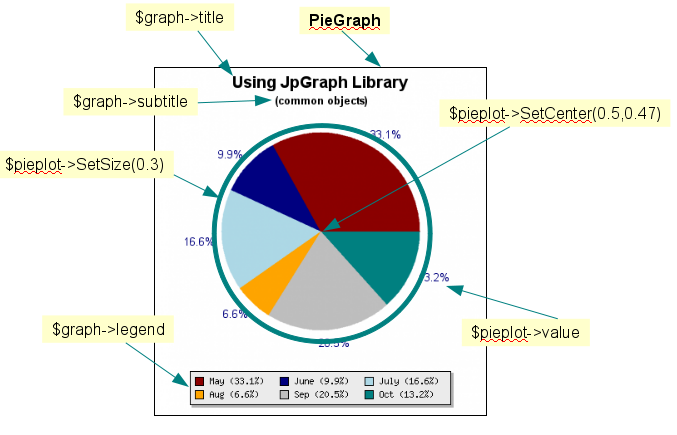## 12.2. Common object for Pie Graphs

For pie plots there are no concepts of axis or scales. Instead Pieplots have a size and position within the graph. Figure 12.2 shows the common objects for a typical Pie graph

Figure 12.2. Commonly used objects in Piegraphs (``` common-obj-piegraph.php```)The following should be noted.

• Instead of an instance of `class Graph` and instance of `class PieGraph` must be used

 ```1 ``` `\$graph = new PieGraph(\$width,\$height);`

• The title and subtitle has the same semantics as for cartesian graphs

• The pieplot is an instance of the `class PiePlot` which is given the data to be used in the pieplot as first and only argument

 ```1 ``` `\$pieplot = new PiePlot(\$data);`

• The size of the pieplot radius is given as fractions of the minimum of the width and height of the graph

• The position of the center of the pieplot is given as factions (width, height) of the graph

• The legend is controlled in the same way as for cartesian graphs by accessing the instance variable "`legend`". In the Piegraph in Figure 12.2 we have changed the layout of the legend by the two lines

 ```1 2 ``` ```\$graph->legend->SetPos(0.5,0.97,'center','bottom'); \$graph->legend->SetColumns(3);```

These two method calls adjust the position and the internal layout of the legend. The first line instructs the library to place the legend at a point (defined in fractions of width and height) (0.5, 0.97) and align that point with the (center, bottom) anchor point in the graph box. The second lines specifies the number of columns to use int the legend.